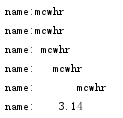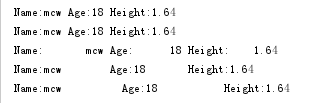python其他知识目录

1、循环打印“我是小马过河”

while True:
print('我是小马过河')

#4、用while从一打印到10

#5、请通过循环，1 2 3 4 5 6 8 9 10

#备注：连续的对其中一个不做相同的操作（连续数字指

#自我注解：无限循环，死循环用while。

if type(变量) == 数据类型：（str ,list,tuple,dict）
print（数据类型）

#4、用while从一打印到10

#注意Python似乎不能用i++,可以i+=1

count=0
while count<10:
count=count+1
print(count)                 #从1到10，（0,10）先自增

count=0
while count<=9:
count = count + 1
print(count)                #从1到10，（0,9]先自增

count=0
while count<10:
count +=1
print(count)               #从1到10，（0,10）先自增，自增可以count=count+1;count+=1；如果自增为一个变量，那么count+=变量

count=1
while count<11:
print(count)
count = count + 1       #[1,11),先其它操作后自增

count=1
while count<=10:
print(count)
count = count + 1      #[1,10]先其它操作后自增

#给自己的建议：程序需要从几到几，按照第一种方式，先自增后其它，实现循环次数后再做操作，自增放在前面防止思路弄乱，方便自己阅读。
#需要添加倒着循环的

#5、请通过循环，1 2 3 4 5 6 8 9 10

#备注：连续的对其中一个不做相同的操作（连续数字指

count=0
while count<10:
count=count+1
if count != 7:
print(count)

count=0
while count<10:
count=count+1
if count == 7 :
continue
print(count)

print("""
1
3
4
5
6
8
9
10""")

count=0
while count<10:
count=count+1
if count == 7 :
pass
else:
print(count)

6  8-10

#6、while else 结构

count=0
while count<10:
count = count + 1
print(count)
else:
print("不再满足while的条件时，即whil返回值为False时执行这里，break等跳出循环的操作是否

#自我注解：循环（正常）结束才执行的代码，非正常结束不执行代码
#添加：上面为循环做完了做什么操作，添加一个循环做完了然后做满足某种条件的才做某种操作，否则是另一种操作。（使用flag）

#7、格式化输出

1、s%  指定占位符宽度

name=["mcw","小马过河","魔降风云变"]
print("%s和%s"%(name,name))
--------------结果：
mcw和小马过河

print("name:%s"%("mcwhr"))            #指定占位符宽度
print("name:%3s"%("mcwhr"))
print("name:%6s"%("mcwhr"))
print("name:%8s"%("mcwhr"))
print("name:%12s"%("mcwhr"))
print("name:%8.2f"%(3.1415926))
--------------结果：print ("Name:%s Age:%d Height:%.2f"%("mcw",18,1.64))                    #加-加数字，可实现几列对齐排序
print ("Name:%-s Age:%-d Height:%-.2f"%("mcw",18,1.64))
print ("Name:%10s Age:%8d Height:%8.2f"%("mcw",18,1.64))
print ("Name:%-10s Age:%-8d Height:%-8.2f"%("mcw",18,1.64))
print ("Name:%-12s Age:%-12d Height:%-12.2f"%("mcw",18,1.64))
-------------------------结果：name=["mcw","小马过河","魔降风云变"]
print("%s和%s"%(name,name)
-------------结果：
SyntaxError: unexpected EOF while parsing （分析时出现意外的EOF）

2、%d        #取整数

print("You have %d books"%(23.4))
------------结果：
You have 23 books

%d可以取整，而%s却不能取整
print("You have %s books"%(23.4))
--------------结果：
You have 23.4 books

3、%%     #打印一个%号，要用另一个%转义，不要被%s的%所影响。

print("我有%s%%的把握"%(80))
-----------------结果：

4、%f     #打印浮点数，以及指定打印小数位位数

print("我支付宝只有%f的余额"%(250.25))
--------------结果：

print("我支付宝只有%.3f的余额"%(250.25))
-------------结果：

#%f可以打印出后面的浮点数，似乎是6位小数。而%s写的啥就输出的啥
print(
"我支付宝只有%s的余额"%(250.25))
--------------结果： 我支付宝只有250.25的余额

name = input('姓名：') do = input('在干什么：') template = "%s在教室，%s。" %(name,do,) print(template)

template = "我是%s，年龄%s, 职业%s。" %("mcw",73,'讲鸡汤',)

print(template)

template = "我是%s，年龄%d, 职业%s。" %("mcw",73,'讲鸡汤',)

print(template)

name = 'mcw'

template = "%s现在手机的电量是100%%" %(name,)

print(template)

占位符%d既可以表示整数int,还可以表示浮点数float(去除整数部分)

占位符%f既可以表示浮点数float,还可以表示整数int(默认保留6位小数)

注意:若想自主保留n位小数,可将其表示位%.nf

pycharm改解释器版本

#8、打印1-100的奇数

count=0
while count<100:
count=count+1
if count%2 != 0:
print(count)

#自我注解：除以2取余为0的是偶数。条件可得偶数，需要奇数，条件取反就得奇数。

#9、求和1-100

count=0
sum=0
while count<100:
count=count+1
sum=sum+count
print(sum)
#注解：count自增实现循环，sum将每次循环的count加到sum里，实现count1-100的循环求和

#10、计算1-10中，除1外，偶数减奇数

zongshu=0
num=1
while  num<11:
if num==1:
zongshu=zongshu+num
elif num !=1:
b=num%2
if  b==False:
zongshu=zongshu+num
elif b==True:
zongshu=zongshu-num
num=num+1
print(zongshu)

#14、数据类型转换

bool() 数字和字符串转布尔类型，0和空字符串是False,空格是True.布尔值转换数字为0和1，转换为字

#需要增加数据类型转换

#15、value=1 or 9  （and,or,not）

#注意：似乎由此判断变量的值，然后对值进

"""

#注解：or遇到假，往后走，遇到第一个真，就返回这个值。遇到全为假，返回最后那个值 $找真找到就停v1= 0 or 0 or False v2=0 or False or 0 print(v1,v2) View Code and """ 对于and，如果遇到 value= 1 and 9 这种情况 如果第一个值转换成布尔值是True，则value=第二个值。 如果第一个值转换成布尔值是False，则value=第一个值。 如果有多个and条件，则从左到右依次进行上述流程。 #注解：and遇到假就停，返回假的值。没有假，返回最后一个值$找假，找到就停

v1 = 1 and 9
v2 = 1 and 0
v3 = 0 and 7
v4 = 0 and ""
v5 = 1 and 0 and 9
j_str = "v1=%s v2=%s v3=%s v4=%s v5=%s" %(v1,v2,v3,v4,v5)
print(j_str)

#v1=9 v2=0 v3=0 v4=0 v5=0

and 遇到0就0，遇到空就空，都么遇到就是最后那个，同时遇到谁在前就是谁？

unicode现在基本ecs4,小表情ecs4,ecs2不包括 gbk gb2312,其中一个是另一个的升级，多一点。二者用两个字节存储汉字。 很多开源组件是国外的，用的是utf-8，建议用utf-8的编码

posted @ 2019-03-28 19:10  马昌伟  阅读(318)  评论(0编辑  收藏  举报